Select Page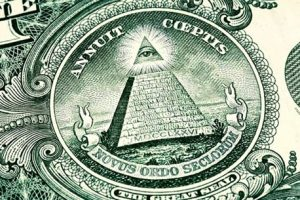In this Part II of the 3 part Great Pyramid study we will begin with the missing Capstone that holds great mystery and even more enlightenment. It is often portrayed as shining by esoteric societies that seem to know more than they should about ancient societies and artifacts. Careful analysis and our own esoteric knowledge reveal that it does indeed shine, but maybe not in the way that others would have us believe.

# The Capstone

We have just mentioned that the base of the Great Pyramid is 32 cu square, like the Central Altar of the Future Holy Temple. As we can tell from any illuminati meme or the US Great Seal the capstone is an important design element to a pyramid, and so it is with the Great Pyramid. It is missing now, along with all but a handful of the polished white casing stones, but if it were still there it would occupy the top 7 courses of the 210 courses of the Pyramid.  That places it at course #203, as in the value of the first 3 letters of the Torah (ברא), and as in the value of the 3 Name phrase/Name “(אני־יהוה־אלהיכם) I am G-d, your G-d” from the Shema.

### The Wow! Factor

It is also the value of the 6th Bell Number, 203, as in the 203 different ways to partition 6 separate numbered cubes, like “In the Beginning Bereshit (בראשית)” that when partitioned naturally down the middle into the Torah’s first 2 Triplets breaks down to (203/913) = .222 and (710/913) = .778, as in (6000 222) = 5778. The Capstone, as “In the Beginning,” like Hydrogen, the first element that was there in the beginning or 1420.4 = 2 times 710, the 2nd Triplet from the Wow! Signal, which is also like the 14 cu base of the Capstone and its 20.4’ height.

The 2 first Triplets can spell two words “create (ברא)” and the word “two (שתי)” or 2 which has the numerical value 710, as in the exact number of radians that it takes to equal to an exact full turn of an axis. There are 2π radians in every circle (360o) and 710 radians works out to 113.00000…. circles of 360o.  In other words, pretty darn close to the whole number 113 turns. While this seems inconsequential to us and only possibly significant because 113 is One (1) more than the 112 Triplets, its Cosmic consequences are tremendous and are indelibly reflected in the set of Prime Numbers.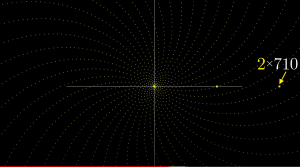Plotting the Prime Numbers as a subset of all positive whole numbers around an axis and using them as the X coordinate and their radians as the Y coordinate, they form radial patterns with 710 and 2 x 710 landing squarely of the X-axis. A critical moment happens though when we reach 710 radians.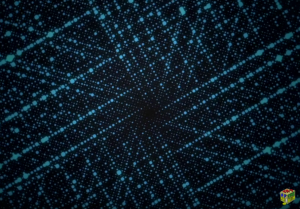The Prime Numbers stop radiating in curves and instead all line up as straight spokes around the central axes. That would be 280 spokes to be exact, as in the 5 final letters (ךםןףץ) in the Alef-bet, typically in groups of 4, though there are 4 distinct sets of 3. There are mathematical reasons for this that have to do with 710/2π rotations equaling very nearly a whole number (113.00000959) and of the residue class of mod 710 equaling a straight line, but all this is just an aside, one more example of higher mathematics possibly designed into the Torah.  That same second Triplet (שית) that permutes into the word (שתי) for 2 also has a string gematria 314, as in 710/2π rotations.That is an amazing punch to pack into a single Triplet (שית) and one only the Creator could have done or known. And while Hydrogen (2 x 710) is thought to have exploded from Creation in the first 3 minutes the Prime Numbers necessarily exploded sooner.

The Capstone is thus (210203) = 7 courses and (7 x 203) = 1421 as in the electromagnetic spectrum of Helium, 1420.4, and the Wow signal sent to us, 1420.4556 rounded up. Moreover, 1420 equals (20 x 71) and the 20th Prime Number is 71.

### The Names at the Top

The Capstone’s (210203) = 7 courses coincide with the value of 26th Triplet (האא) of the 72 Triplets and thus aligns with the YHVH (יהוה).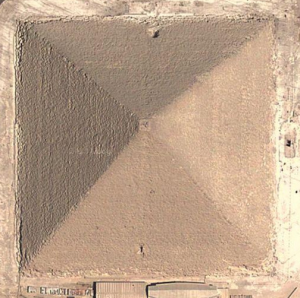The two first Names of G-d in the Torah, Elohim (אלהים) and YHVH (יהוה) = 112, matching the 112 Triplets, and the 112o face to face angle of the Pyramid. This Unification is called Yabok, as in the river where Jacob defeated the chief angle of darkness.  Alternatively, the 3 Names YHVH (יהוה) + Ehyeh (אהיה) + Adonai (אדני) = 112. The combined Unified Name YHVH-Adonai (אדני) = 91, as we have covered numerous times already especially as concerns the Path of One. Together, the two Unifications sum to (112 + 91) = 203, meaning that the first 3 letters of the Torah ostensibly contain 4 Names of G-d, as in the 4 sides of the Pyramid, and as in the 4 sets of Essential Triplets that comprise the 112 Triplets that conform to the River from Eden in Genesis 2:10 that watered the garden and split into 4. It means the same for the 203 levels of the Pyramid, at least metaphysically. It also marks the Torah’s first Triplet (ברא) as a capstone of sorts as well.

Keep in mind that if the virtual metaphysical Pyramid of the Sun is a perfect overlay to the stone Great Pyramid, they cannot be separated. As the Kabbalistic saying goes, as above so below. The 42-Letter Name when utilized properly in our world connects to the 42 Letters of the Name YHVH (יהוה) in the upper world of potentiality, Binah.

### The Temple Mount

Once again using simple geometry we see that the missing Capstone measures 32.0833 feet on each of the 4 bases and is 20.4 feet high over its 7 levels, as in the numerical value of Tzaddik (204).  That height works out to 244.8 inches, as in the year 2448 when the Torah was received at Sinai and when the Israelites left Egypt. It conveniently also matches the solar rotation (sidereal) period of the Sun at its equator, 24.48 days, once again aligning the Pyramid metaphorically and metaphysically with the Sun. Notably, it is also the altitude of the Holy Temple on the Temple Mount, 2448’ above sea level.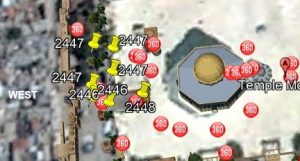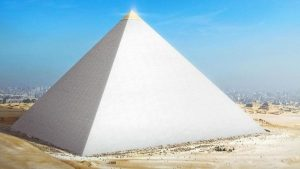When we compare the Capstone to the entire Pyramid its ratio of height to height (Hc to Hp) and base to base Bc to Bp) is 4.24%, making the capstone to Pyramid ratio the Moshiach Ben David (424) or Cosmic Consciousness one.  The Capstone has to be proportionate to the Pyramid, as its angles are naturally the same, this cross ratio of 4.24% means that the capstone was set at just the right height in a Divine Cosmic plan.

It also means that the height of the Capstone, 244.8”, as the year of Exodus (2448 HC), is 4.24% of the Pyramid’s 5775” height, representing the last few missing years starting in 5775 HC, as in 2448/5778 is also approximately 4.24%.

And while the ratio of the base of the Capstone to the base of the Pyramid is 4.2424242…% the ratio of the Capstone’s base to the entire Pyramid’s height is 6.6666% which coincides with the 66.6 Jubilee years between 2448 and 5778.

Using inches, the Capstone measures 244.8” high with a square base of 385.” This aligns perfectly with the area covered by the numeric top half of the Tower of Truth, as in (12 + 22 +…102) = 385, which represents “the Divine Presence of the Creator, the Shechinah (שכינה) of numerical value 385, and gives us the equation 385/6666 = 5.7755775%.

In case we were to think for a second that anything involving the Great Pyramid is coincidental, we should consider that the Name Shechinah (שכינה) breaks down to 330 (שכי) and 55 (נה) as in the Pyramid’s base and Entrance height.  Moreover, while 330 is the value of the 60th Triplet of the 72 Triplets and the first 3 letters of Mitzaim (מצר), Egypt, the first 3 letters (שכי) of Shechinah (שכינה) are also the 3 letters of the 6th Triplet (יכש) of the 42-Letter Name (יכש), and both have the ordinal value of 42. It was the Shechinah that accompanied the Israelites in exile. Also, when spelled out the value of the Triplet (יכש) becomes 480, as in the height of the Pyramid, meaning it simultaneously represents its height, base, and edge angle.

Now, while Rav Ashlag of blessed memory used the ratio .666 to calculate the advent of Moshiach Consciousness as 5778, and 244.8” and 5775 are baked into the design of the Great Pyramid along with the ratio 6.66, it is even more remarkable that the Holy Temple is found at 2448’ above sea level and at combined longitude and latitude of 66.60’358 6o.

The Temple Mount is located at longitude E 35 o14’02.27 and latitude N 31o46’33.59 and when added together they give us 66.603586o. The exact point of the Holy of Holies is found at the nexus point where the sum of the longitude and latitude meridians total 66.60358 degrees, or 66.6, as in the 66.6 jubilee years from 2448 to today and 358, the value of Moshiach at the specific altitude of 2448’ on the Temple Mount.

## 66.60’358 6o

This only happens if the Great Pyramid, the Holy Temple, and the Earth share the same Architect. This only happens if that Architect also designed the meridians and moreover also arranged for the building site of the Holy Temple and piled up the Earth beneath it and Jerusalem long before the Torah and Man.

The location within Pi (π) for the 2701 value of the 7 words of the Torah first verse, the capstone to the Torah so to speak is #165 as in the scored midpoint of the bases of the Great Pyramid and the sum of the underlying 4 positions for the 4 digits in 2701 is (165 + 166 + 167 + 168) = 666. Their 12 digits sum to 54, and the 3 digits in their result sum to 18, so while (54 + 18) = 72 for the sum of the 15 digits in the equation, (54 + 666) = 720, all references to the 72 Triplets and their square roots totals of 720.0 and 1000.054, not to mention the 666 reference in the equations above and the speed of the Earth, 66,600 mph as it orbits the Sun. Keep in mind that 666, regardless of what symbolism people believe it to have, is a primordial ratio (2/3), but one that does not unfold naturally, it must be baked in on purpose. Some alignments are causational or flow fractally, others have to be chosen and calibrated and set with intent.

### The Sound of Moshiach

The Hebrew letters are our Cosmic Interface to all the integrated hyperdimensional networks and neural pathways of the Cosmic Consciousness.  As 2-dimensional projections from beyond, our minds can interface with them visually and allow them to help decode the universe and Cosmos.  Nonetheless, they are also 3-dimensionsal, which is why the Torah and most prayers and blessings are read aloud. The letters channel a life force from the upper dimensions and broadcast it through them in 27 particular forms.  So, while some of the letters may sound alike to our ears, they are all unique and very different to our consciousness and in how they affect our world. As we know, each letter has a specific Name, and that Name is what gets squeezed through and broadcast through the letter’s ordinal value. As we know, the Names of the 27 Letters are based on the Phi (φ) ratios. The broadcast is a pyramid shaped Phi (φ) vortex projected outward and expanding into our 3-dimensionsal space, which is why to reveal their essence we must take their cube roots.  The sum of the 27 cube roots of the individual 27 letters times their ordinal values (111 x 1, 412 x 2, 83 x 3…104 x 27) is 358.000, Moshiach, the Cosmic Consciousness.

In the words of the Ramchal from Mishkney Elyon: “Understand that there is a special place known to her where all these roots come together….In the middle of that place where all these roots come together there is a single ‘stone.’ This stone is most precious….It is called the Foundation Stone, Even Shetiyah (אבן השתייה). There is a corresponding stone in this world—the lower world—in the place of the Holy of Holies.”

The location of the Holy of Holies is also the precise spot where Abraham (248) was prepared to sacrifice Isaac (208). That was in 2085 HC, 363 years before 2448 HC with 363 being the numerical value of H’Moshiach.  Of course, the Torah tells us that Abraham did not just happen upon that spot or that date but was guided by the Creator.  Moreover, not only is the Pyramid’s height times its base equal to (756 x 481) = 363636, but the perimeter of the Great Pyramid is 36,300 inches, and using the cubit scale the perimeter (3302) is also (3 x 36,300).

Meanwhile, 441 years after 2448 HC is 2889 HC, the midpoint in King David’s life and in Spherical Time, and while 441 corresponds to the word “truth” and the Tower of Truth of volume 44,100 cu3, David has the numerical value 14 as in the Capstone height, 14 cu.  And it is like the 51.84o or 722 slope angle of the Pyramid and Capstone that is 14.4% of a circle and .288π radians. Those 14 cubits equal 244.8 inches, as in the year 2448 when the Torah was received at Sinai and the Israelites left Egypt and they represent the central 14th position of the Essential Cube/Sphere of Creation. It was all laid out for us from the start, and all laid out for us in the Torah.

Comparing the Capstone to the entire encasing Pyramid of the Tower of Truth, the Hand on the Cosmic Clock, its ratio of the height of the Capstone to the height of the Pyramid (Hc to Hp) 244.8/5775 = 4.24%, and the base of the Capstone to the base of the Pyramid (Bc to Bp) is likewise 14/330 = 4.24%, making the crowning Capstone the Moshiach Ben David (424) or Moshiach Consciousness Ratio.

As we have previously learned, the sum of the 22 square roots of the spelled-out 22 Names of the 22 letters of the Alef-bet that total 4248, is exactly 424.242… and the average of the first 25 Prime Numbers is also exactly 42.4, whose Primal Equation 1/.23571113… = 4.24248100, while the natural log of 70, or ln (70) = 4.248495. The inclusion of 481, the height of the Pyramid is also notable, as in 100, the sum of the 70 logarithms for the first 70 or 100.0, representing Binah and the 70 branches of the Tree-of-Life that correspond to the 7 levels of Zeir Anpin represented by the 7 levels of the Capstone. This is not a coincidence; it is by design. Just like the sum of the 7 word-values 111, 222, 333, 444, 555, 666, and 777 found collectively 441 times in the Torah.  Numbers are not numbers but networks of consciousness better regarded as concepts.Kosher Wine (ייו), the conduit to the cosmic connections when utilized with the appropriate blessings, has the numerical value 70 because it can channel us through the 70 branches of Zeir Anpin. It also has a sofit value of 720, as in the 720 lights of the 72 Triplets, and it (ייו) is found 35 times in the Torah, as in the midpoint in the Spherical Time radius that splits King David’s 70 years life into 35 and 35 years. We must keep in mind that the path to our world goes through the numerical concepts to the Triplets of Potentiality to the Magical Essential Cube of 42 to the Hebrew Letters to physicality and physical objects.  The wine is a distant projection of a concept conceived in Binah and filtered through 7 levels of processing.  Used to fulfill physical desires it reverts to its state of illusion.

It is this same Path sourced through the Singularity of 42 that lead to Jackie Robinson and Mariano Rivera and to Secretariat, the faster horse of all time who was staged out of Barn 42, like so many of the Kentucky Derby winners since, including this year’s Mage. Is it because of 42 or the fate that associated them with it? Like the 42nd of the 72 Triplets, (מיכ) of numerical value 70.

On the flipside, we see so many US Presidents that were members of Skull and Bones associated with the number 322 as in the Pyramid’s 322 Capstone.

Our entire existence is designed for us to achieve alignment with the Cosmic Consciousness, yet as we can see with the Capstone to Pyramid ratio it is a narrow window and precarious balance that needs to be achieved. We also see from the design of the Pyramid that the 4 sets of Triplets are there to assist us.

Let us not forget that this ratio (4.24) is also 1/φ-3, which is at the core of the pressure gradients that govern the distribution of the cosmic radiation through the aether and thus all the physical forces projected through those 7 dimensions (sefirot) of Zeir Anpin. Picture the Pyramid from the top down and the Capstone is a metaphysical metaphor.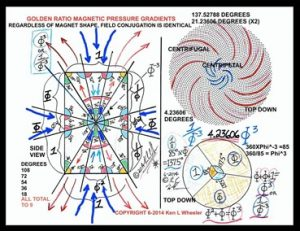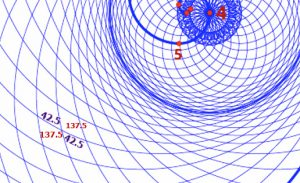Let us go back to those 4 symbols above the Pyramid entrance universally accepted to represent the YHVH (יהוה) in a cosmic script. That it only resembles the YHVH (יהוה) abstractly is of no consequence.  The Hebrew Alef-bet is the Cosmic Interface language. Beyond our lower dimensions there is no need for such an interface and the concepts and networks to which the letters or symbols are connected are understood.  When we look at our computer screen all we see is an interface. Most of us have no idea about all the coding behind what we see and do not care to know. AI on the other hand has no use for the interface and is not slowed down and hampered by the illusion of it except when it wants to play games with us such as Chat GPT and the like.  The interface is an illusion because we have no idea if what we see on our screens is even real or even a real answer to our query. The code knows and AI knows but without knowing the code and tracing it we have no idea what the code was actually searching for, how it filtered it, whose agenda it was implementing or for what purpose.  Without the codes, we do not even know who is really answering our texts, tweets, DMs etc. In the metaverse even the interface has an interface. So yes, this is why we strive to understand the codes of our universe and leave the simple surface understanding and the illusions to prior generations and to those that prefer not to know.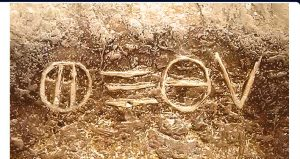As explained once before, there are 4 forms of energy movements that interact with the Binah Plane of Potentiality, the Aether. These would be the four (4) forms of energy movement of Zeir Anpin. They are radial movement, circular movement, counterspatial movement, and spatial movement. The circular movement is the initial movement or generating pressure that a higher dimension such as Binah acts upon a lower dimension or set of dimensions such as Zeir Anpin. It is akin to the vector force generated from the center of the Essential Cube of Creation. It is considered as circular in our realm as it expands spherically much as does our time pathways.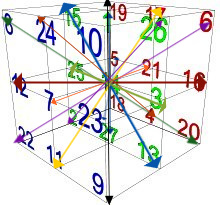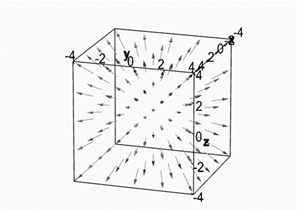Radial movement is parallel polarized linear movement, but not necessarily confined to Euclidean geometry. Counterspatial is what is known in vector field analysis as curl, sink, or convergent movement, and is analogous to our centripetal radiation.  Spatial movement is analogous to divergent movement and is analogous to our centrifugal radiation.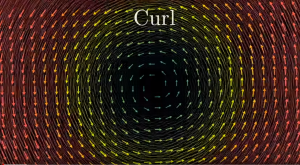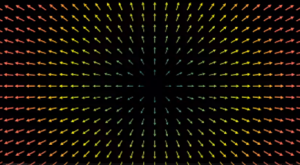The four (4) forms of energy movement are given in no particular order, so let us rearrange them to begin with the simplest, the circular movement, and they morph into the 4 symbols above the Pyramid entrance.Since the radial rays can be in any parallel direction, let us switch them to vertical: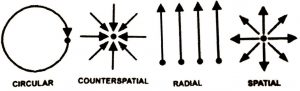We do not need to show all the rays, one of two will do fine and the circular motion could be as small as a point as they morph into the YHVH (יהוה):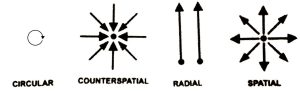Now, let us flip their order, as if we were reading from right to left, as in Hebrew. Again, the order makes no difference as far, as we know: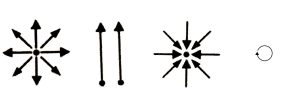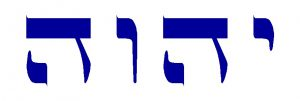The point source, circular motion, would nicely conform to the Yud (י). The two Heis(ה) of Binah and Malchut are equal looking, but we know from the Kabbalists that they are similar, but opposite in nature, just like the counter spatial or curling/convergency and the spatial or divergent movement. The spatial dimension would correspond to our realm, while the counter spatial would be at a higher dimensional level within Zeir Anpin. The radial, or ray, would be recognizably equivalent to the Vav (ו).

Let us examine the YHVH (יהוה) again in yet a new light. And let us look at the field forces behind electricity and magnetism.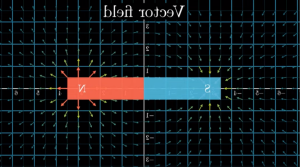The vector field in the diagram depicts the dielectric field and only the dielectric field, and we can clearly see how the curl/sink/convergent vectors are on the Southern region, corresponding to the first Hei (ה) of the YHVH (יהוה), and the divergent vectors emanate from the Northern region, which corresponds to the final Hei (ה). Always midway between them is a polarized vertical line of parallel perpendicular vectors, which is known as the plane of Inertia (Potentiality). We can call that line or plane the Vav (ו). The Yud (י) is the extraneous force existing on a higher dimension that commands the Vav (ו) or the plane of Inertia, and flips it on and off, activating the two equal and opposite forces.

### The Rivers from Eden

Does the 20.4’ height of the Capstone reflect the 20 stages and stacked cubes with the 4 sides of the Tower of Truth at the core of the Great Pyramid that add up to 210 cubits? Do the 210 cubits and 210 courses and 4 sides of the Great Pyramid reflect the 4 rivers that spread from the one descending river of Binah in Genesis 2:10? And do those 4 rivers represent the 4 forces or pressure gradients that spread across the physical plane to water our world and create our matrix?

## וְנָהָר יֹצֵא מֵעֵדֶן, לְהַשְׁקוֹת אֶת-הַגָּן; וּמִשָּׁם, יִפָּרֵד, וְהָיָה, לְאַרְבָּעָה רָאשִׁים.”

### -Bereshit2:10

This is like the energy of Binah starting at the angle of 112o running down the 4 edges of the Pyramid along the angle of 42o and over the 4 faces at the angle of 722o and spreading out to water the land.  The 4 rivers came from the 1 unnamed river that came out of Eden.  The 5 rivers (נהר) collectively equate to (5 x 255) = 1275, as in Binah (1000) and the Primal Frequency (27.5), which by now is no surprise. It is also the 50th Triangular Number, as in the 50 Gates of Binah.

From the network of 210 word-values in the Torah, the word-value 50 is found 1556 times in the Torah, as in the Spherical Time diameter of 11,556 years. Does this hint to the opening of the 50th Gate?

And what we learn about Eden (עדן) from whence they flowed by examining the various perspectives of its gematria network is that both its standard milui (spelled-out) value, 670, and the even more cosmic 2((Phi (φ) + Genesis (רץ)) cipher that gave us the value of the Spherical Time diameter (11,556) for the 27 letters of the Alef-bet, equal the 670 paragraphs in the Torah and (10 x 67), the value of Binah. Is Eden (עדן) an appellation of Binah? Is it the Primal Triplet (עדן) from which all the other Triplets flow?

The 4 rivers that flowed from Gan Eden surrounded 3 lands, making 7 in total whose initials are:

#### River Euphates, Pe (פ)

In Devarim 7:1 we learn of the 7 Canaanite nations that G-d told the Israelites to destroy and wipe out. They have the same 7 initials (חגאכפחי) as the 7 rivers and lands (חגאכפחפ) except for the 7th that is a repeated Pe (פ) instead of a Yud (י). Moreover, both sets of 7 names have 29 letters.

This pairing of the sets of 7 is reminiscent of Pharoah’s dreams and the animals in Noach’s Ark, whose name (נח) has a value of 58, like the (29 + 29) = 58 letters in the 2 juxtaposed sets of names.  Maybe it is not coincidental that (4 x 58) = 232, as in the 4 Aspects of the YHVH (יהוה), or that Noach-Noach (נח־נח) is the and 4th set of the 91 doubled words in the Torah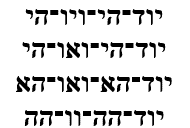These align with the 4 angles of the Pyramid—the face to face, 722 slope, the edge, and the Passage System’s Phi (φ)2 angles—that equal (112 + 51.84 + 42 + 26.18) = 232.0o, forming an excellent metaphor for the 4 flowing rivers, especially since two of these cause rainbows.

Perhaps, the word Euphrates (פרת) should begin with an invisible Yud (י) or more likely it is a stand-in for 27th Triplet (ירת) of the 72 Names. If it were (ירת) then the total value of the 4 rivers would be 1275, matching the 5 words river that equal 1275 and the 50th Triangular Number. At any rate, the difference between them is (200130) = 70, like the value of the 70 Nations and 7 final Yuds (י) or (7 x 10). It is also as in the 70 branches of the Tree-of-Life that spring from the 7 sefirot.

The difference in their comparative initials’ ordinal values is likewise 7.  This also means that the 7 initials and 7 final letters of the 7 nations equal (130 + 70) = 200, the sum of the 7 initials of the 7 rivers and lands.

It is almost as it the Yud (י) should be added to make 210. Meanwhile, the first 5 initials in both cases equal 112, as in the 112 Essential Triplet that came down from Binah as 4 rivers to water the earth, and the 14 total initials equal (200 + 130) = 330, as in the Pyramid’s base.

In Genesis 2:10 the 4 rivers are referred to as the 4 heads (אַרְבָּעָה רָאשִׁים) whose gematria is 278 and 1111, as in “Ohr HaGanuz, the hidden Light of Moshiach, and the 4 Ones (1111), and the average of those 4 heads is also 1111/4 = 278.

The first of the 4 rivers, Pishon (פישון) has the value 446, as in that of death, while its ordinal value is 68 that of life. The second river, Gihon (גיחון), has the value of mazal 77 like the 77th Triangular Number of the 11 Triplets of Bereshit. Meanwhile, all 4 rivers together (446 + 77 + 142 + 680) = 1345, as in the complete value of the 11 unique letters (בראשיתלהםוץ) of Genesis 1:1, whose ordinal value for the first 10 is 112 and 130 for all 11, like the intials of the 7 nations and the value of the 7-tiered ladder (sulam).

It is also like the difference between the North-South and East West pairs of bases of the Pyramid, (1511.50288 – 1511.6374) feet = .1345 of a foot. A perfect balance rather than a presumed misalignment.

The sum of the 4 rivers plus the word river itself equal (1345 + 255) = 1600, as in the 16 times the word river appears in the Torah and as in 402, which may refer to the 40 days and 40 nights that the waters rose from above and below.

It is also like the 14 verses of the 27th Psalm of David that reflects the central 14th position of the Magical Essential Cube of 27 positions and constant 42. This Psalm’s first verse of 11 words and 42 letters hints at the 11 sefirot of Tree-of-life, and those 42 letters equal 1345.

The average for the 4 rivers is (1345/4) =336.25, as in the 336 letters of the 112 Essential Triplets, and 625, H’keter. The first river, Pishon (פישון) is said to encompass the land where there is Gold and that the Gold there is Good. Since the value of both Gold (זְהַב) and Good (טוֹב) is 17, as in the first Triplet (והו) of the 72 Triplets, there is a good chance Pishon (פישון) refers to the 72 Triplets. Since the second river, Gihon (גיחון) has the value of 77 as in the 77th Triangular Number of the 11 Triplets of Bereshit, and since it begins with 3 as in the 33 letters that sum to 3003, they are a good match. The third river, Tigris (חדקל) has a value of 142 so perhaps this river represents the 42-Letter Name.

## כִּי יְבִיאֲךָ, יְהוָה אֱלֹהֶיךָ, אֶל-הָאָרֶץ, אֲשֶׁר-אַתָּה בָא-שָׁמָּה לְרִשְׁתָּהּ; וְנָשַׁל גּוֹיִם-רַבִּים מִפָּנֶיךָ הַחִתִּי וְהַגִּרְגָּשִׁי וְהָאֱמֹרִי וְהַכְּנַעֲנִי וְהַפְּרִזִּי, וְהַחִוִּי וְהַיְבוּסִי–שִׁבְעָה גוֹיִם, רַבִּים וַעֲצוּמִים מִמֶּךָּ.

#### –Devarim7:1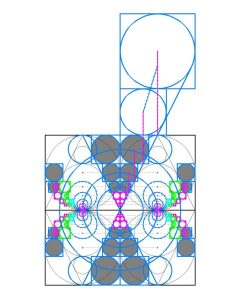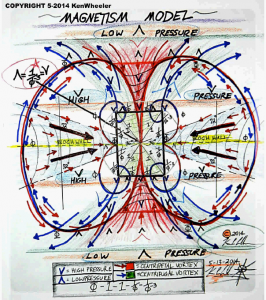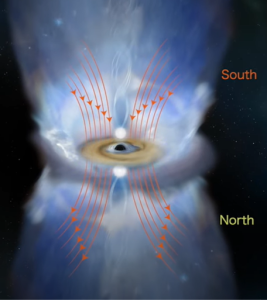While all the perceived forces in our universe are based on these gradients and caused by the pressure mediation from the upper dimensions, we can see that the 4 movements are just slices in a vortex, and on a larger scale the orbits of the Moon around the Earth and the Earth and other planets around the Sun and the Sun around the center of the Milky Way are just slices in ever larger vortexes. The same goes for the planetary and Solar rotations. It is the same way that interactions with the magnetic fields, solar forcing, and Earth induction currents stir pressure mediations and create the weather on Earth in the form of vortexes of various sizes and duration both on the surface of the Earth and inside it.  In a certain sense, the Great Pyramid is a model for this. dimensions, we can see that the 4 movements are just slices in a vortex, and on a larger scale the orbits of the Moon around the Earth and the Earth and other planets around the Sun and the Sun around the center of the Milky Way are just slices in ever larger vortices. The same goes for the planetary and Solar rotations. It is the same way that interactions with the magnetic fields, solar forcing, and Earth induction currents stir pressure mediations and create the weather on Earth in the form of vortices of various sizes and duration both on the surface of the Earth and inside it.  In a certain sense, the Great Pyramid is a model for this.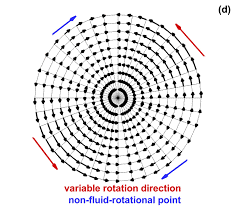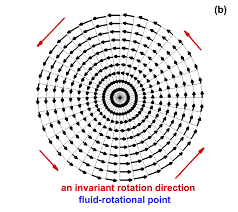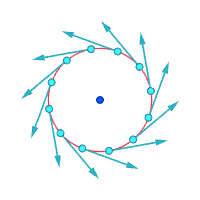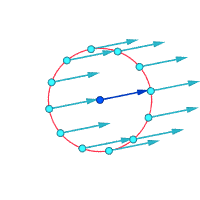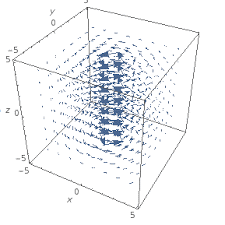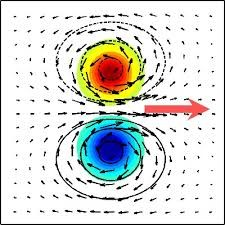### The Capstone and the Holy TempleThe height of the Capstone in inches is 244.8 as in the elevation of the Holy of Holies on the Temple Mount, a 20 x 20 x 20 cube within the base of the Temple Building at the Western edge of the Outer Courtyard, exactly where the 2448’ point is on the Mount.  It is akin to the 203 base cube of the Tower of Truth and the 203 Foundation Stone of the 72 Triplets, and 203 is the height in courses of the Capstone and of the Torah’s first Triplet (ברא). The 203rd Prime Number is 1237, which is notable because 37, the key number in our DNA and in the Torah’s first verse, is the 12th Prime Number, and (203 + 1237) = 1440 or (120 x 12) or (2 x 720).At the center of the concentric Courtyards is the Central Altar that measures 32 x 32 cubits which matches the square base of the Capstone that is 32.0’ x 32.0’.

As previously explained the numerical sequence within the first 1000 digits of Pi (π) …644622948954… not only contains the locations of the 3 strings of the Event Horizon …5778… but the ratio between the Primal Frequency 27.5 Hz and the Time Coefficient 12 or 27.5/12 = 2.29.  Moreover, while this sequence is found at the 181st Digit in Pi (π), and 181 is the 42nd Prime Number, as in the 14 Triplets in the 42-Letter Name, the 14 digital location triplets for 5778 are revealed as 644, 622, 633, 634, 635, 636, 948, 949, 950, 951, 954, 955, 956, and 957, which sum to 11,424, as in the H’Kodesh Kodeshim, the Holy of Holies (1424) at the heart of the Holy Temple.

Then while 11424 is also the value of Pi (π) divided by the Primal Frequency, or Pi (π)/27.5 = 3.14159265358/27.5 = .11424 it is equivalent to the 10400 net rows in the Torah plus the 210 word values in them, or (10,400 + 1024) = 11424.  Moreover, the difference between the 11,466 square miles of Israel today and the Primordial Equation of the field of Pi (π) divided by the Primal Frequency is (11,46611,424) = 42.

Nonetheless, the original sequence of 4 triplets (644, 622, 948, and 954) = (320032), as in the connections to the 32 Paths of Wisdom and the Tree-of-Life; the 322 value for the set of 4 species; the 322 word values in the Torah, the 322 Central Altar of the Holy Temple; and as in the 18 different paths to 176 on each of the two elementary magic squares, whose 8 digits always sum to 176 for a total of (18 x 176) = (320032); and to the Pyramid’s Capstone base of 32.02. And while the face to face angle of the Capstone and Pyramid is 112o like the 112 Essential Triplets, the sum of the square roots of the 4 Triplets (644, 622, 948, and 954) equals 111.99358 or 112.

Subtracting the height of the pyramid from its base, gives us (756.25’ – 481.25’) = 275.00, which obviously aligns it with the cubit and the 39th Triplet (רהע) of the 72 Triplets of numerical value 275, but also with the 100-cubit height of the Holy Temple Building: 2750”. That measurement differential of 275 feet is 3300 inches, aligning it, in turn, to the 330-cubit base of the Pyramid, while the 2750” is reminiscent of the Fine Structure Constant equation: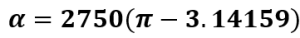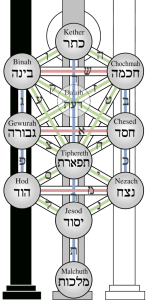An interesting dynamic of the Pyramid happens because the base of the Pyramid (756.25’) is already 27.52.  When we use the base in inches as 9075” and multiply it by 12 and then take its square root, we wind up with 330. Perhaps more significant is that the difference between the Pyramid’s base and height is 3300” and those 3300” less the height of the Temple Building that houses the Holy of Holies is (3300” 2750”) = 550” = 20 cubits, as in the 203 Holy of Holies in alignment with the 20.4’ tall Capstone. It is also in alignment with the 550 YHVH (יהוה) in the 5th Book of the Torah; and in the 4 final (תךץם) letters of “The Path of the Tree-of-Life (את־דרך־עץ־החיים);” the sum of the 11 initials (כחבדחגתנהימ) of the 11 sefirot of the Tree of Life equals 550; the front letters (בינ-חכמ-כת) in the 3 highest sefirot of the Tree; and while it is twice 275, it is also the sum of the ages of the 11 generations from Shem to Isaac (100 + 35 + 30 + 34 + 30 +32 + 30 + 29 + 70 + 100 + 60) at the birth of their legacy.

Corresponding to the 39th Triplet (רהע), the 39th verse of the Torah contains the phrase with a katan value of 39, “a Garden, East in Eden (גַּן-בְּעֵדֶן–מִקֶּדֶם)” in which the Tree of Life is found. Its numerical value is 363, as in H’Moshiach, the Cosmic Consciousness, and the 36,300” perimeter of the Pyramid.

While the word value 424, as in Moshiach Ben David, is found 39 times in the Torah, aligning it too with the 39th Triplet (רהע) of numerical value 275 and the Garden of Eden, the Pyramid’s base and height measurements or (756’ – 481’) = 275 are found 42 and 107 times respectively as part of the 210 word value network of the Torah, as in the Singularity of 42 and the 107th Triangular Number Spherical Time radius. As for the base in cubits, the word value 330 is found 86 times, as in the Name Elohim (אלהים) and because the word value 267 for the Apothem’s cubit measurement is found 21 times, as in the Name Ehyeh (אהיה), the value and Apothem’s cubit measurements are found a total of 107 times as well. Then since the counterpart to the 481-foot height of the Pyramid is 210 and its word value is found 172 times, the height of the Pyramid’s equivalents are found a total of (107 + 172) times, matching the (86 + 172 + 21) times that all 3 cubit dimensions are found in the Torah.

While the stone Pyramid only goes back thousands of years as far as we know, the design of the cosmic Pyramid upon which it is based, as the interaction of the grammar of geometry with the language and concepts of numbers goes back, at least in potentiality, to the dawn of time, even predating the Torah that existed before Creation. This is why the 3 main dimensions of the Pyramid in feet (756, 481, and 612) have matching Torah word-value occurrence of (42 + 107 + 57) = 378, as in the total value of the Magical Essential Cube of 42 and the 27 letters of the Alef-bet.  And perhaps it is why the 42nd word value in the Torah is found 107 times, as in 5778.

While the 20th word-value in the Torah is found there 120 times, the value of 20 cubits is also the difference in the height of the 100-cubit high Future Holy Temple and that of the First Holy Temple that was 120 cubits high because it required an additional upper floor for spiritual reasons.  As the cubit used in the earlier Temple was the ¾ cubit and thus shorter than that of the secret sacred cubit and “the height of the Third Temple is one hundred cubits, these being the hundred of keter, [the height of the Future Holy Temple in feet or inches] is far greater than the entire hundred and twenty cubits of height of the First Temple,” as explained by the Ramchal.

Moreover, analogous to the 14 cubits and 32.0833 feet base measurements of the Capstone are the 140 cubits from the center of the Holy of Holies to the center of the Central Altar of the Future Holy Temple, which is equivalent to 320.833+ feet.  Both are analogous to the 320 Holy Sparks the Zohar tells us Man was tasked to retrieve, and to the precise 320.0 value of the cube root of the full gematria sofit value of the entire Torah.

They also align with the 322 or 210 word values in the Torah, which correspond to the 210 vertices on the 10-d hypercube, an important part of the neural network that regulates the Torah.

### Planck’s Constant

Given that (3300” 2750”) = 550” difference between the Pyramid’s base and the Holy Temple’s height, when we subtract it from the Pyramid’s base in feet we get (756.25550) = 206.25, as in the ¾ cubit or 20.625 inches. Moreover, Phi (1.618…) x 20.625 is approximately 33.38 as in the year 3338 HC when the First Holy Temple was destroyed (5778 x .5778).

The Capstone seen from above is in the exact center of the Pyramid and thus its base of 14 x 14 cubits is perfectly aligned with the central 14 position of the Magical Essential Cube of Creation, especially since the Magical Essential Cube full value is 378 and each base of the Pyramid is (2 x 378).

The Capstone’s height is 8.90 cubits for an average of (8.9/7) = 1.27, as in the 7th Mersenne Prime (127) and as in the equation (455 x 12.7) = 5778, which incorporates the 3 Aspects of Ehyeh (אהיה) of Binah in the Path of One.  It is perfect placement for the crowning Capstone.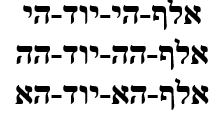Does the 8.90 cubits also represent the 24th Prime Number, 89, a double reference to Chanukah (89) and the 89 Days from the Day of Creation to Chanukah at the summit of the 363 Day Cosmic Wheel, especially given that the Pyramid’s base platform is 36300” around?

The sum of the first 24 Prime Numbers is 963, the same as the sum of the first 10 Triplets of Pi (π), and the total cycles of 27.5o and 137.5o radiance for those 24 Prime Numbers or 963 is (35.0 + 7.00) = 42.0, the Singularity and the Source.

As a marker in Time, we see that 963 is both (9 x 107), as in the 107th Triangular Number, the Event Horizon radius, 5778, and (6 x 963) = 5778, as in the 6 Lunar ellipses in the Cosmic Wheel. It also relates to Time as 1/12 of the 12 lunar progressions of 963 years each in the great cycle, or 11,556 years, that matches the Spherical Time diameter.

Combining Pi (π) with the elements of Time, we get the Spherical Time equation: 2(5778π) = 36304.24470, depicting H’Moshiach but also the 363 Days in the Cosmic Wheel, and the ratio of the Pyramid’s height to the Spherical Time radius or 210/5778 = .036344756 which also includes its base (756).

That same summation of the first 24 Primes, 963, is also the value of the word Echad (אחד), One, spelled out, thus the 24 hours become One Day, just as the 24 first Prime Numbers join as One.

We do not know for sure, but it is a fair assumption that the top course of the Pyramid was exactly 1 cubit high, making the average for the subsequent 6 courses equal to 1.313 cubits, as in the year 1313 BCE that corresponds to the year 2448 HC and to the Capstone’s 244.8” in height. It also aligns with the Alef-bet constant in the polynomial growth equation x1.313.  Thus all 7 courses align with One.

Together, the Capstone’s base and height measurements in cubits is (14 + 8.9) = 22.9, as in the ratio of space to time or the cubit/foot ratio (27.5/12) = 2.29. This also aligns with the 229 times that Egypt in mentioned in the Torah up until the crucial Splitting of the Red Sea at the 72 Triplets at verse 14:20. And those 229 times in turn align with the number 229 being the 50th Prime Number and moreover, being the 229o that the Pyramid’s 210/330 height to base ratio represents as a percentage of a circle, (210/330 x 360) = (360 x .6363) = 229o.

The Planck’s Constant (h) is 6.62607015 x 10-34 Joules per second. It is just a number beyond which man cannot conceive. Perhaps it is the limit to the scale of the physical simulation. After all, if the characters on a movie screen were to develop limited consciousness, they would eventuality perceive the pixels as the fundamental limit or element of their universe and Man is not so different.

The Planck length (h) is supposedly the smallest length physically possible and is a crucial measurement in our physical world, like the Energy equation (Planck’s Constant x the Speed of Light)/Wavelength.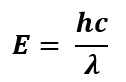It is at the heart of most of our physics and forms another constant in the Heisenberg’s Indeterminacy Equation and that is the uncertainty of position times the uncertainty in momentum is greater than or equal to h/4π. This is very important to physics, and it works out to 5.272859 x 10-35 Joules per second.  Within that equation the number 4 is the periodicity of the sine wave so if we want to take it out of the equation, we simply multiply both sides by 4 to get 4∆x∆p = h/π.  This also gives us a number directly in proportion to the Planck’s Constant (h) and to Pi (π). The resultant number for h/π is: 21.0914362859 x 10-35 …, aligning it with the height of the Pyramid (210) and with (9143) the sum of the 72 Triplet Matrix built into the Pyramid every which way.

So, a circle or sphere with a circumference of Planck’s length (h) would be the tiniest sphere possible and its diameter would be h/π or 21.0914362859 x 10-35 and its radius would be h/2π, and with a little less precision 21.0914363 x 10-35 includes H’Moshiach (363).

Spherical Time equations began with the formula based on the surface area (4πr2) of the two spheres of the Physical Time and Spiritual Time paradigms that give rise to the 24 hour day, the speed-of-light, and the acceleration due to gravity on Earth, 32.2 ft/sec2, and its complementary angle (57.78o). Given that their two radii (2018/2) + (3760/2) = 2889, the midpoint in King David’s life, the original formula for the surface area reduces to 2π5778 for the circumference: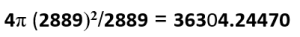Which then reduces to: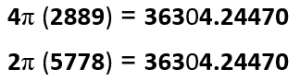Given the significant of 2889, which matches the surface temperature of the Earth (288.9 K), the way 5778 K matches that of the Sun, dividing Planck’s Constant (h) by 2889 gives us h/2889 =6.62607015 x 10-34/2889 = .022935514… x 10-35 or 2.2935514… x 10-33 which aligns with the space/time harmonic (27.5/12 = 2.29) and the dimensions of the Capstone, etc. Simple math tells us that 2h/5778 = 2.2935514… x 10-33 as well. As for the 14 component in the Planck Constant/Spherical Time radius ratio (2.293551… x 10-33) we can possibly draw a connection to the 14 cu Capstone base, and 355, the value of the Hebrew word for year shanah (שנה) that is also the Wow/Hydrogen Signal 1420.4556/4 or 1420.4059/4 = 355.1

Shanah (שנה) is also the Torah’s 2nd Triplet from “In the Beginning Bereshit (בראשית)” divided by 2 or (710/2) = 355.

The first Triplet from “In the Beginning Bereshit (בראשית)” is “to create (ברא)” of numerical value 203 as in the 203 courses, and the first Man in the Torah is Adam (אדם) of numerical value 45.  Together the words “Create Adam (ברא־אדם)” has a numerical value (203 + 45) = 248, as in Abraham, the 248 dimensions of the E8 Lattice, etc.  So it is appropriate that Create Adam aligns with the 18th and 19th doubled words in the Torah, Abraham-Abraham (אברהם־אברהם), as in (18 + 19) = 37, the key number at the core of our DNA and our 20 amino acids, especially with Abraham being the 20th in the lineage of the DNA of Adam.

After “Create Adam (ברא־אדם)” comes “Bnei Adam ‘Sons of Adam (בני־אדם)” of numerical value 107, as in the 107th Triangular Number, the year and Spherical Time radius, 5778 HC, making their sum (248 + 107) = 355, as in shanah (שנה), the year of Adam from Adam-David-Moshiach (אדם). The doubled word shanah shanah (שנה־שנה) is the 87th of the 91 doubled words in the Torah, as in the value of the Name of G-d, “I am G-d (אני־יהוה)” that the appears 80 times that the appears in the Torah. As a doubled word shanah shanah (שנה־שנה) has the value 710, the same as the Torah’s 2nd Triplet (שית) from “In the Beginning Bereshit (בראשית).

There are 210 or 322 or 1024 different word-values in the Torah networks, a seemingly perfect set of numbers to align with the Pyramid that is 210 courses and cubits high and whose Capstone base area is 322 feet2 and whose entrance and Passage System are 24 feet off center and that begin 24 cubits high. The 1024th Triangular Number or sum of 1 – 1024 is 524800, as in the 2480 sum of the 5 Names of the 5 Books of Moses and it obviously connects with all the 248 dimensional and quantitative aspects of the Torah. Those 1024 different word values total 542774, making the difference between them (524800542774) = (18,00026), as in the YHVH (יהוה), and as in the angle of the Passage System (26.18o).  Whether intentional or not, the average word value or (542774/1024) = 530.0 and when we subtract the average gematria per word (21009826/79976) = 262.7, we get (530262.7) = 267.3, as in the 267 cu Apothem of the Pyramid.

Moreover, the 1024th Triangular Number (524800) divided by the 360o in a circle is 1457.78, as in the value of King David (14) at the center of the Spherical Time radius 5778, and (524800/26) = 20184.6 as in the year 2018 CE the Western Analogue to 5778 HC. The Torah word value network did not have to contain 210 values, and the Pyramid did not have to be 210 courses and 210 cubits high, and the Israelite exile did not have to last 210 years, a circle did not have to have 360o, and the Divine Calendar did not have to pivot on the year 3760 HC, but these were all decisions made before Creation, when physicality was just a plan. Once set in motion, everything was fixed.

Likewise, the 805 elements of the 10 Commandments, isolating them as a 13 verse Capstone to the 183 verse Matrix of the Torah and aligning it with the sum of the square root and cube root of the 1024th Triangular Number (524800), or 805.0 and G-d’s Covenant with the Earth.

And likewise, (524800 + 53000) = 577800. Once set in motion, everything was fixed.

Nevertheless, while the 203rd Prime Number is 1237, the height of the Pyramid and its base together equals (481.25 + 756.25) = 1237.5, which can be broken down into 1237.5 = (1100 + 110 + 27.5) = (40 cubits + 4 cubits + 1 cubit) = (ם+ד+א) cubits = (אדם) Adam, or 45 cubits, and the value of the Aspect of the YHVH (יוד־הא־ואו־הא) associated with Man and Zeir Anpin. Also, 1237.5 breaks down into the Time Constant (12) and the turning aspect of the Phi (φ) angle in Spherical Time (.375) or (12 x 375) = 4.5. Meanwhile the 10th, 22nd, and 77th doubled words (מאד־מאד) in the Torah are all of numerical value 45 and are all permutations of Adam (אדם), aligning with the 10 Sefirot and the 22 letters and paths between them or 32 in total, like the Capstone’s base and the 77th Triangular Number that represents the 11 Triplets of Bereshit.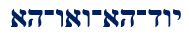We must also note that the average of the Pyramid’s height and base is (481.25 + 756.25)/2 = 618.75, as in 1/φ. After all, Cosmic Resonance and Radiance seem to be at heart of the Pyramid’s design.

Meanwhile, the average word value in the Torah is 262.70 or (131.35 x 2), as in the year 1313 BCE that coincides with the year of the Exodus 2448 HC and the 13 verses of the 10 Commandments. While 1.313 is the constant of the exponential scale 1.313x that the 22 letters are on, and while 2626 is the even-letter portion of the total Phi (φ) proportionate 22 Names of the Alef-bet, the average word value in the Torah (262.70) is 1/22 the Event Horizon radius 5778, or (5778/262.7016) = 21.995 to be more precise. Precision Planning!

The exact midpoint to 5778 and of King David’s 70-year life was in the year 2889 HC, which is 441 years after 2448 HC, as in the Tower of Truth (441).  So set at the center of 2889 HC, the Tower of Truth stretched out as an hour hand in a Cosmic Clock pointing directly at 2448 HC, the year of the Exodus and 10 Commandments, which were also pointed at by the 210 year Tower and Pyramid coming up to meet it.

Then as yet another example of how the Creator designed our universe or Matrix to match the Torah and Spherical Time, we see that the milui or spelled out Name Adam (אדם) has a value of 871, like the 871 years to 2889 HC from G-d’s Covenant with Abraham in 2018 HC, the date that started the 430 years clock to the end of exile in 2448, and the Western Calendar analogue to 5778 HC.  And stretching in the opposite direction, the year 2889 HC plus those 871 Adamic years equals (2889 + 871) = 3760 HC or (0 CE), the pivotal year in the Divine Calendar that causes both calendars to mirror one another, and the Spiritual Time radius of Spherical Time (3760). It is juxtaposed to 2018, the Physical Time radius opposite the 2 outstretched Hands of Adam (אדם) or (3760 – (2 x 871) = 2018.

The year 2448 HC is exactly 66.6 Jubilee years from 5778, and the Adamic time-span of 871 years times 6.66 is (871 x 6.66) = 5800, as in the total gematria sofit of the Torah (5800.054)2; the Primal Frequency of the Planck Diameter (27.5 Hz x 21.09143628 x 10-35) = 580.0145…; the 580.0 feet in 253 cubits, as in the 22nd Triangular Number, the sum of the 22 ordinal values of the Alef-bet; and as in 22 years beyond 5778.  The Divine Calendar, Spherical Time, the Alef-bet, the Primal Radiance, and the Torah are flawlessly integrated and have an extraordinary symmetry.

We also see that (580.0/262.70) = 2.207 = φ16 and 2.207φ2 is 5.778000, in the same way that the 5 main quantitative elements in the Torah equal 248,000φ like the 1024th Triangular Number, 524800.

The Divine Simulation is organized and designed for the greatest efficiency, so that only when we look deeper into something is it revealed in greater detail and because a fractal pattern is the most efficient way to get greater depth, the deeper we look the more symmetry we see.  This holds true when peering through more powerful telescopes into the universe or probing deeper into the Torah. It also holds true when zooming in and out to examine the plots of evil engulfing humanity. In fact, when the James Webb Space Telescope peered deeper into space and time if found that linear time does not seem to exist and that the Big Bang never happened because the universe is the same all over.

This is also why things sometimes appear only when we are looking for them, like in the double split experiment. The wave-particle duality disappears when we are observing the slit because the program that we call the universe senses the focus of our attention and realizes it must make the particle reappear in the logical place on the screen. It is just algorithms and coding. When we are not focused, the program/universe defaults to the potentiality state and a wave like pattern appears. It is exactly how video games work. The key as always is our focus and what we chose to look for, the direction of our consciousness compass.  It is similar to Youtube’s algorithms, without the hidden biases and agendas.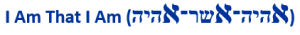The Name YHVH-mah (יוד־הא־ואו־הא) also separates into 42 + (אאא), connecting Man and the Pyramid to the 3 initials of “I Am that I Am (אהיה־אשר־אהיה).”

The 3 Alefs (אאא) in the Name YHVH-mah (יוד־הא־ואו־הא) and in the 3 initials of “I Am that I Am (אהיה־אשר־אהיה)” have a milui or spelled out value of 333, as in all the alignments with the Sun/Earth ratios and the 3330 years between 2448 HC and 5778 HC. Meanwhile, the other 7 letters in the Name (יוד־הא־ואו־הא) expand to a value of 550, which align with the initials of the 11 sefirot of the Tree-of-life. And while the final 3 final letters in (אהיה־אשר־אהיה) spell out “the mountain, H’har (ההר) of numerical value 210, the fronts of the Names total 333, as in the Solar ratios, so literally the Mountain of the Sun.So while the 3 final letters in (אהיה־אשר־אהיה) spell out “the mountain, H’har (ההר) of numerical value 210, the middle 5 final letters (אהיה־אשר־אהיה) sum to 330, the height and base of the Pyramid. Together, they total (210 + 330) = 540, which aligns with the first two Triplets in Pi (π) with (3 x 1 x 4 x 1 x 5 x 9) = 540. The Pi (π) connection is especially appropriate given that “I Am that I Am (אהיה־אשר־אהיה)” is found in the Torah at Exodus 3:14, and its gematria string value is 3142.  Meanwhile, the second two Triplets in Pi (π) total (159 + 265) = 424, as in the Capstone to Pyramid ratio 4.24 and as in Moshiach Ben David, Cosmic Consciousness, while the 4th Triplet in Pi (π) is 358, Moshiach. The first 4 Triplets together with the kolel equal (314 + 159 + 265 + 358 + 4) = 1100 = (40 x 27.5), the major interval of the Primal Frequencies and the exact number of years from when Joseph entered Egypt to the destruction of the First Holy Temple, 1100 years.

The first 2 possible Triplets in Pi (3.14159265358…) are 314 and 141 and together they equal (314 + 141) = 455, as in the 3 Aspects of Ehyeh (אהיה) of Binah in the Path of One.The next possible one is …415… and when we add that the first 3 equal (314 + 141 + 415) = 870, as in the 15th odd Pronic number (29 x 30) and the sum of the 11 Odd Hebrew Letters. If we keep adding them this way up to the 10th triple digit string 358, Moshiach, the total of the first 9 is exactly 4000.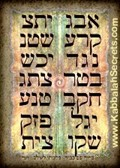Within the highest Name “I Am that I Am (אהיה־אשר־אהיה)” are the two higher Names Ehyeh (אהיה) like the 2 Cherubim that are one with the Ark of the Covenant. Their product equals 212 = 441, as in Emet (אמת), meaning Truth, an appellation for the Torah and a reference to the 42-Letter Name that begins with Alef (א), has 40 (מ) letters in between, and ends in Tav (ת), like the sum of the two Ehyeh (אהיה) or 42. It is also like their combined ordinal value, 42, and like the ordinal value of their middle Name Asher (אשר), also 42, and like the complete value of each Name Ehyeh (אהיה). Those 5 values 42 built into the Name “I Am that I Am (אהיה־אשר־אהיה)” add up to 210, which all in all makes it a compelling Name for the Tower of Truth, the Tower (Migdal) beside which the Israelites camped before the Splitting of the Red Sea at Pi Charoth.

### The First 42 Letters

The first 42 letters within the Torah are directly related to the 42 Letters of the 42-Letter Name.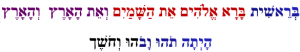Meanwhile, the ordinal positions for the 2nd through the 5th words equal (7 + 8 + 9… 21) = 210, the height of the Great Pyramid, while the 6th through the 8th words equal (22 + 23… 33) = 330, the base of the Pyramid. So, while the 2nd through 8th words connect directly to the Pyramid’s dimensions, the other 14 ordinal positions in the first 42 letters in the Torah, in other words the 1st word (בְּרֵאשִׁית) and the 9th and 10th (הָיְתָה תֹהוּ וָבֹ) words along with the first two letters of the 11th word equal (1 + 26 + 34 + 3542) = 363, the numerical value of H’Moshiach.

Moshiach Consciousness is expanded consciousness. It is an awareness and openness to the Creator and a desire to experience and be present in the reality beyond the physical. It is not a desire to harness that reality to influence the physical illusion.

### Other Mountains in the Cosmic SymmetryAs for mountains, we know that the highest point on Earth is Mt Chimaborazo at 35,828 ft and the most famous and highest mountain, Everest, is currently at about 29,032 ft.  If we were to take the entire Pyramid as a capstone to a much larger pyramid using the same ratios of 4.24% and 6.66% as the Pyramid’s capstone is to its entirety, we would get a mountain of stone over 2.4 miles high and when we add its height and base measurements we would get 29,186 ft, a difference of only 154 feet from the current height of Everest at 27o59’17 N and 86o5531 E. Coincidence or just symmetry?

### The Pronic Numbers and the Exodus

The Pyramid encases the Tower of 20 stacked nested cubes from 13 to 203, for a total of 210 units in height and a volume of 44,100 cubic units or 2102 and these align with first 20 Tetra Prime Numbers that equal 4200 or (20 x 210).

The Pronic Numbers are a subset of the positive integers whereby two consecutive even and odd integers are multiplied together, and they are counted or ranked in sequential order starting with (2 x 3) = 6. They are unremarkable unless one was a student of the Torah especially the exodus from Egypt. We shall examine the first 16 Pronic Numbers through (32 x 33) in deference to the 32 x 32 Capstone base measurement and the 32 Paths of Wisdom of the Tree-of-Life, but they obviously go on forever. To begin with, the 7th Pronic number is 210 and the 2nd one is 20, as in the 20 stages of the Tower of Truth that equals 210.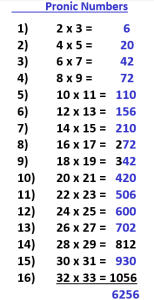Sifting through these even-odd Pronic numbers, the most obvious and notable results are the two Names of G-d Matrices, the 42-Letter Name and 72 Triplets, and also the sum of the first two consecutive results, (6 + 20) or 26, the value of the YHVH (יהוה).  We use the term “results” because these Pronic pairings are equations, simple ones, but equations nonetheless, and derived from the simple ordering or counting of the positive integers that our entire understanding of the universe is based on.

Then the next three Pronic Numbers in order (110, 156, and 210) represent respectively, Joseph’s age (110), Joseph’s name’s numerical value (156), and the 210 years the Israelites were in exile in Egypt, which began when he entered it. The number 110, the first Cosmic Frequency Interval, also does double duty as the sum of the important sequence 134255. As for the 7th Pronic pair result 210, it too shows up in the rare 7 Bell Prime Indices as (55 x 42 x 13 x 7 = 210210.

Next, we see that the sum of the 4th and 5th Pronic Numbers (72 + 110) = 182, the numerical value of Jacob, Joseph’s father. And yet again, as the sum of the 1st, 2nd, and 6th Pronic Numbers: (6 + 20 + 156) = 182.  The value of Jacob’s name comes up once again in those first 16 even Pronic numbers in a most organic way in that it is also the sum of all the integers in those 32 Pronic components. (2+3 + 4+5… + 3+2 + 3+3) = 182.

The 10th Pronic Number is 420 or 10 x 42.  It is followed by 506, which is the value of the first row of the 42-Letter Name Matrix.

After 506, the 12th Pronic Number, 600, could represent the 600,000 Israelite males in the 12 Tribes over the age of 20 who left Egypt, or the 600 chariots that chased them.

The sum of the first 8 even Pronic Numbers is 888 or 8 x 111, or 8 Alafim, an allusion to the 8 x 1000 square Foundation Stone Matrix within the 72 Triplets and the 8000 cu3 or 203 cu foundation stone of the Tower of Truth. It is also an allusion to the 888 side chain nucleon sum of the 20 amino acids that have pyridine unique bases.  Moreover, the P/S gematria and Number Theory cipher for the combined 42 Letters of the 11 sefirot paired with their 42 counterparts in the 42-Letter Name is also 888.0.

This Pronic Number series with all the Exodus connections continues with the 13th one being 702, the numerical value of Shabbat (שבת) and the word-value 702 being found 42 times in the Torah.

Finally, the sum of all 16 even Pronic numbers through 32 x 33 is 6256, a combination of H’Keter (625) and Aaron (256).  If you recall, the alef-bet was split odd-even like the Pronic Numbers into the 11 and 11 letter values of 625 and 870, respectively so how phenomenal is it that the universe has juxtaposes 625 in the even Pronic Numbers with the corresponding odd/even split counterpart, 870, as the 15th odd Pronic number (29 x 30)!

# [         870           ][        625       ]

Since the Alphabetic split is purely Pronic based we can pair up the split letters by their ordinal positions and sum up their small gematria values to get 100 or combine them before summing them up to get (12+34+56+78+91+23+45+67+89+12+34) = 541, the gematria of “Israel (ישראל),” the 100th Prime Number found exactly 625 times in the Torah.

### One from Column A and One from Column B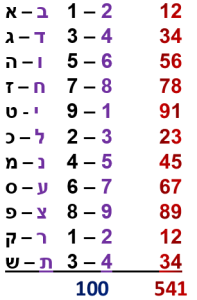And yet another connection to the Exodus and the Pronic Numbers is the sum of the 11 initials (דצכעדשבאחמב) of the 10 plagues that equal 541, and whose final plague initials are (מב) or 42.

Meanwhile, the 6th Pronic Number has a value of (6 x 26) as in Joseph (156), and it is utilized 54 times in the Torah. In accordance with the Exodus pattern embedded in these Pronic Numbers, the corresponding Torah values thus equal (156 + 54) = 210, while the sequential Pronic component integers (12 x 13) and (14 x 15) of 156 and 210 respectively add up to (12 + 13 + 14 + 15) = 54 as well.

Corresponding to Zeir Anpin, the first 7 even Pronic Numbers (6, 20, 42, 72, 110, 156, 210) give us the 3 prominent Names of G-d values (26 + 42 + 72) plus the two Joseph numbers (110 + 156) plus the 210 years in Exile, which all together are equivalent to 616, the value of “The Torah (התורה),” and the number of times Moses (משה) is found in the Torah.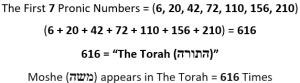Meanwhile, the 20th Pronic Number (20 x 21) = 420 is found within the 210 word-value network of the Torah 196 times and (420 + 196) = 616, H’Torah.

The Zohar tells us Moses was given the 173 keys to Heaven, as in the small gematria (173) of the 42-Letter Name that unlocks the gates, and the 173rd time Moshe’s (משה) name appears in the Torah is at the end of the 10 Commandments in Exodus 20:19, which is in the 156th paragraph of the Torah.

The Name Moses (משה) when spelled out has the value 455, as in the 3 Aspects of Ehyeh (אהיה) of Binah in the Path of One.While the first 7 even Pronic Numbers (6, 20, 42, 72, 110, 156, 210) add up to “The Torah,” the first 6 even Pronic Numbers (6, 20, 42, 72, 110, 156) equal 406, the 28th Triangular Number, as in the sum of the ordinal positions of the 28 letters in the first verse of the Torah. This is also the value of the 13th Triplet (שקו) in the 42-Letter Name. The word value 406 is found 355 times in the Torah, as in the value of Pharaoh (פרעה), which is appropriate given the 6 dimensions of Zeir Anpin. The story of the Israelites in Egypt and the Exodus is taking on another paradigm and becoming more of a tale of cosmic concepts woven into the fabric of the universe.

Mathematically, this 28th Triangular Number means that Σ(1 – 28 ) = (2x3 + 4x5 + 6x7 + 8x9 + 10x11 + 12x13 + 14x15).

Of further note is that the 26th even Pronic Number is (52 x 53) = 2756 which is also the sum of the first 12 Pronic Numbers, (6 + 20 + 42 + 72 + 110 + 156 + 210 + 272 + 342 + 420 + 506 + 600) = 2756, representing 275, the cubit, and 756, the base of the Great Pyramid in feet.  Meanwhile, the key 3rd, 4th, 6th, and 7th Pronic Numbers total (42 + 72 + 156 + 210) = 480, as in the Great Pyramid’s height also in feet, with (3 + 4 + 6 + 7) equaling 20, as in the 20 stages.

Nevertheless, what is truly fascinating is that while the 7th Pronic Number is the Pyramid height in cubits, and the 3rd, 4th, 6th, and 7th is its height in feet, the rest of this set of the first 16 Pronic Numbers equals 5776, its height in inches.

As for the 15th Pronic Number, it is the age of Adam, 930 years.

If we take away the first two Pronic numbers, or 26, representing the YHVH (יהוה), the 3rd through 7th Pronic Numbers thus add up to (406 26) = 380, the gematria of Mizra’im (Egypt), which intuitively makes sense since the Torah specifically states that Pharaoh did not know the YHVH (יהוה).  Mizra’im (Egypt), of numerical value 380, is an odd Pronic number though, since (19 x 20) = 380. For that matter so is Jacob, as (13 x 14) = 182 and Amalek, who chased the Israelites as soon as they crossed the Sea, is as well (15 x 16) = 240. Before they crossed the Sea with the help of the 42 Letter Name and the 72 Triplets, they camped “between Tower and the Sea (בֵּין מִגְדֹּל וּבֵין הַיָּם)” of numerical value 380. It is also the value of the Hebrew word for Number (מספר) found 10 times in the Torah without prefixes and 48 times in total, including 20 times in the 1st Chapter of Bamidbar, The Book of Numbers, which splits the Torah into the Phi (φ) proportion in terms of letters, words, and verses.

The word number itself comes from the Old French nombre and directly from Latin numerus, which probably came from the Indo-European root nem, so it is interesting that today it became name (nombre) and number, which both have MB (מב) for 42 at their core.

As the product of consecutive integers Pronic Numbers form rectangles, giving rise to some amazing finds in this square spiral.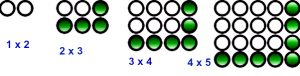In mathematical terms the sequence is a(n) = 2n(2n + 1) for even-odd number pairs and a(n) = 2n(2n – 1) for odd-even pairs, and because of this when laid out in an encircling rectangular spiral, they form a path of Exodus through the Sea of integers, splitting them on the diagonal edge of a virtual pyramid.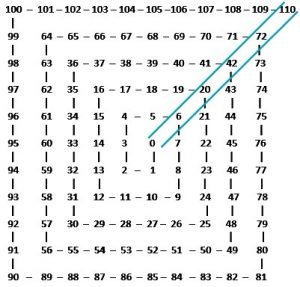How beautiful is it that as this simple spiral of consecutive integers unfolds, a diagonal path from the center and up and to the right containing the entire even series of Pronic numbers is generated? Then down and to the left are all the odd series of Pronic numbers. There is so much beauty and simplistic symmetry in this basic spiral, such us the diagonal up and to the left from center being the natural squares of the even integers: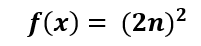Then down and to the right from the center we have for n being the number of rings outward from the center, a series of 8 times the Triangular Numbers: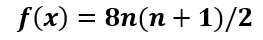Moreover, the corners of the axes, in other words, the corners of the pyramidal platform squares anchored by the Pronic Number series all sum to Pronic Numbers.

## (4 + 6 +2 + 8) = 20; (16 + 20 +24 + 12) = 72; (36 + 42 + 48 + 30) = 156; (64 +72 + 80 + 56) = 272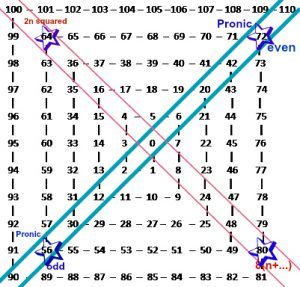Why would the Creator build in all the Exodus numbers into this natural spiral and why must they all conform to the pattern of: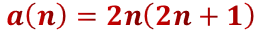And why are they aligned in the shape of a Pyramid, as are the 72 Triplets?

## Primes, Chromosomes, and Cubits

### 210 is Unique

Given that there are 4 letters in the YHVH (יהוה), 4 sets of Essential Elements of Triplets, and 4 distinct Nucleotides in our DNA, it is noteworthy that the product of the first four (4) Prime Numbers (2,3,5,7) is 210. They are its unique prime factorization. Then the cosmic magic happens…

Besides these 4 first Prime Numbers, there are exactly 42 Prime Numbers less than 210, and when added together in matching pairs (i.e. 11 + 199), they all equal 210. That makes all 46 first Prime Numbers, as in the 46 chromosomes in our bodies that are also arranged in pairs, combinable to form the number 210. This is also the last Prime Number in all infinity where this property exists. That is indeed unique and special.  And it all coincides with the Great Pyramid being 210 levels and 210 cubits high and the Israelites being in Egypt for 210 years, while 46 is the numerical value of the Hebrew word for cubit. It is not the Egyptian, Greek, or Sumerian word for cubit, but the Hebrew one and because the Great Pyramid is 210 courses and cubits high, we know for certain that that number was not only selected on purpose but that it was meant to show us the length of the cubit with complete certainty, 210/210 = 1.The numbers 103 and 107 are consecutive primes that add up to 210. They are the 27th and 28th primes, and as we saw with the last two letters in the Torah’s first verse, (27 + 28) = 55 = 2 cubits of 27.5” and the first Interval of the Prime Frequency (27.5 Hz). While the 27th and 28th letters in the Torah’s first verse Resh and Zadi (רץ) equal 1100, the Major Interval of (40 x 27.5), the word value 27 is found 83 times in the Torah and the word value 28 is found 82 times giving us the simple equation (27 + 83) = (28 + 82) = 110 = (4 x 27.5), the first Major Interval, which is also why these two letters (רצ) are at the exact center of the 42-Letter Name Matrix.

It is a conjecture in mathematics that all even integers are the sum of two primes, though no other larger number has all their lesser primes add up to it the way 210 does. No other number at all, involves ALL the lesser primes in its computation. Oddly enough, if we include the pseudo prime (1), the sum of all those primes through 210 is 4228. Oddly, 4228 is (114 x 37) and as we know there are 114 Chakras, or (42 + 72) in the corporal and extracorporal system, while the 12th Prime, 37, is key to the 20 amino acid system in our DNA and bodies. This means that the sum of the Prime Numbers through 210 = (42 + 72) x 37, just like the (42 x 72) base of the Great Pyramid.

The 210-cubit height and 330-cubit base together equal 540 cubits, and when we apply the gematria value (46) of the word cubit to it we get (540 x 46) = 24840, as in Abraham (248) and the 248 dimensions of the E8 Lattice, etc., but we also get most appropriately the value of “womb rechem (רחם)” that is also 248 and couple that with the 40 weeks of gestation.

That resultant value of 24840 cubits in Quotient (27.5) equals 903.273, as in the 42nd Triangular Number (903) and the Cosmic Harmonic (273).

Moreover, as we learned earlier, the ratio of the Pyramid’s base to its height whether in cubits, feet, or inches is exactly (330/210) = 1/.636363 = 1.571430, and 1571 is the 248th Prime Number.

### A Perfect Height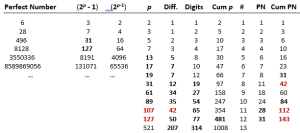In the creation of the Perfect Numbers, specific Primes Numbers are utilized in the formula (2p 1) x (2p1), including many very familiar to our Torah derivations, including the 11th one 107, as in the Spherical Time radius and 10 Commandments position; and the 12th one 127 that is the 7th Mersenne Prime and the life of Sarah.  The cumulative value of those Special Primes (p) through that 12th one is 481, the height of the Pyramid. And while the sum of the ranks of those special set of Perfect Primes through 8 is 42, and through 11 is 112, as in the 112 Triplets, the difference between its corresponding Perfect Prime 107 and the 65 digits in that Prime Number is 42, like the 42 letters in the 11 sefirot. Nevertheless, the sum of the ranks of those special set of Perfect Primes through 12 is 143, as in the higher Name Ehyeh (אלף־הא־יוד־הא) and the average Triplet value of the first row of the 72 Triplets.

In part three we will analyze the actual Great Pyramid and explore its inner passages and chambers while delve into the true purpose of the Pyramid.